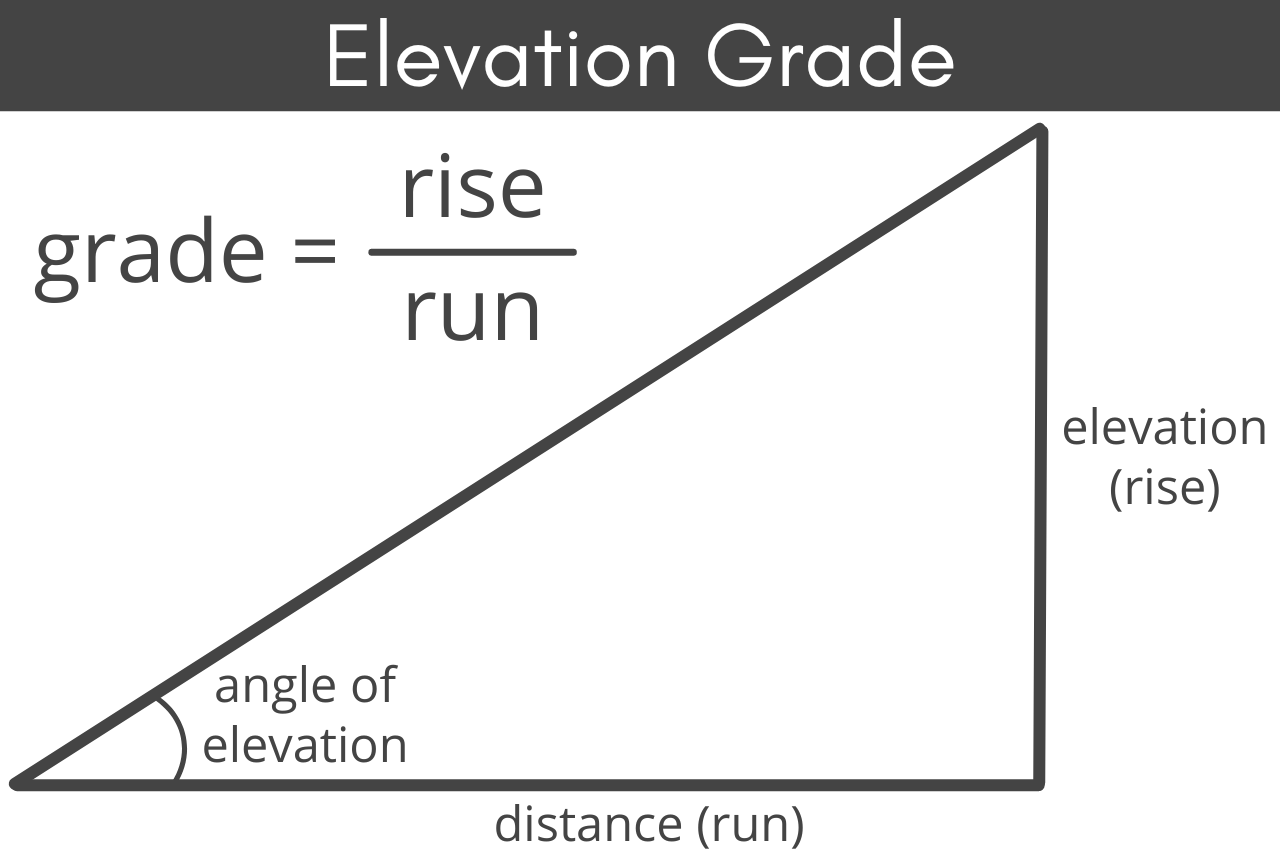Calculate the grade of an elevation in degrees or grade percentage and estimate the horizontal or vertical distance needed to support an incline to an elevation.

## Results:

Elevation

Decimal

Percentage

Degrees
Learn how we calculated this below

Grade, also referred to as slope, is the amount of incline of an elevation, for example, the angle of the side of a hill. Grade can be expressed as the amount of rise over run, as a percentage, or as an angle measurement in degrees or radians.

Grade measurements are often used in projects ranging from roofing to road or driveway construction. They can also be useful in determining drainage when installing a patio, since grades that are too steep can be hard to use, while areas that don’t slope enough may not drain properly.

Grade measurements are also used to ensure that ramps meet ADA requirements and for roof design for buildings. Because roof pitch is calculated slightly differently than most other projects, see our roof pitch calculator to learn more about estimating the slope of a roof.

## How to Find the Grade of an Elevation

The grade of an elevation can be found by measuring the horizontal length of an elevation, also known as the run, and the vertical height of the elevation, or the rise.Grade is expressed as rise/run, so if the rise is 25 and the run is 80, then the grade is 25/80. This can be reduced to 5/16; use our fraction simplifier to help reduce larger fractions if needed.

Keep in mind that both your rise and run must be in the same unit of measurement. For example, if you measure your rise in inches but your run in feet, you will need to multiply your run measurement by 12 to convert it to inches so that your rise and run will match.

Because many grades are shown as a percentage, such as the common 5% grade required for a patio to drain, you will often find that you need to convert your fraction to a percentage. To find the percent of incline of a grade, solve the rise/run fraction as a decimal, then convert to a percentage.

For example, if the grade is 5/16, solve as a decimal by dividing 5 by 16, which gives you 0.3125.

To find the percentage multiply this decimal by 100%, like this: 0.3125 × 100% = 31.25%. For comparison, something held at a 45-degree angle has a grade percentage of 100%.

The angle of incline can also be expressed in other units of angle measurement, such as radians or grads. While this is less commonly needed by homeowners, laypersons, or landscapers, it may be useful for some applications. You can use our angle converter to convert your slope from degrees to one of these measurements.

## How to Measure an Elevation

Whether you are calculating the rise of a slope in your backyard or the slope of your driveway, the measurements are typically done in a similar manner.

### Step One: Measure the Run

First, you’ll need to mark the beginning and ending points of the rise.

This can be done using stakes or simply placing a marker on the ground. Measuring the distance between your two markers to get your run.

### Step Two: Measure the Rise

To measure your rise, you can use a device such as an altimeter to get your exact elevation at each marker. Then simply subtract the elevation at the start of your run from the elevation at the end of your run to get your rise.

In addition to an altimeter, you can also use surveying levels to help you measure the rise. Dumpy levels, transit levels, and laser levels can all be used to help give you an accurate measuring point at the bottom of your rise. Set up your level using a tripod at the endpoint of the run, so that it is level with the top or endpoint, then measure from the ground to the level to get the rise.

However, if you do not have access to an altimeter or surveying levels, you can measure the rise manually.

Place a wooden stake at the end of the highest point in your elevation and tie a string to the top. Walk the string out to the lowest point and hold it level – this can be checked with a manual level or your phone’s level app held gently to the top of the string.

If possible, tie off the string to keep it level, or have an assistant hold it at level while you measure the distance from the ground up to the string. This will give you the rise.

When measuring rise using any type of level – surveying or string – the measurement from the ground to the level is your total rise. For example, if the measurement from the ground to the level is 20 inches, then your rise is 20 inches.

### Step Three: Calculate the Grade

Finally, divide the rise by the run to calculate the grade of the elevation. Then, simplify the fraction if necessary.

The elevation is calculated using common trigonometry formulas; learn more on our angle of elevation calculator.